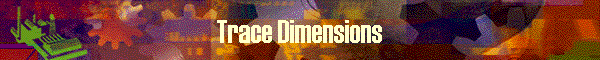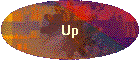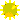This panel shows the history of dimensions in number.

The history is shown under the columns of below;Template    shows the dimension numbers of template rod, or the dimension numbers of compared-to RodID.Previous     shows the dimension numbers which was designed by previous trial (when design was repeated)Latest        shows the dimension numbers which is designed latest time. This matches to the contents of Excel work file and Designer Excel Tools.Bend       shows the bend angles in number which matches to the Latest dimension numbers.  In case the design is made via Stress Curve, this field shows the resulted Bend Angle.RodID      shows the owner RodID of the dimensions of Previous and Latest.Template Rod ID    shows the RodID which is used as template or compared-to.

What is Previous?

On both of [Design by Deflection] and [Design by Stress], there is a choice for users between "start with template" and "repeat on the work file".  If user select "repeat on" at this time, it is possible to iterate the design and adjustment as you like.  The previous field maintains the previously designed taper.

This panel has no update capability on dimension numbers since the both design-by functions themselves intend to design the dimension numbers.

Exactly same dimension numbers are copied into both of Excel Designer Tools at "Data" worksheet.

### An Usage of this Dimension table

[Design by Deflection] and [Design by Stress] program develops the point=0 dimension based on the point=1 dimension by a certain algorism.  This is because the point=0 dimension cannot be calculated by any program logic.  Point=0 dimension somehow affects to the dimensions of entire rod.  Especially it affects to the dimension around the tip top.

In this case, it is a good practice to read the differences of dimensions around tip top, especially point=0 dimension from this table.  Then goes to the panel of [Define Deflection] or [Define Stress] to specify  the possible dimension number on "Tip dimension at point 0" field prior to re-calculate the dimensions.  For this, several trial and error are required.

When the point=0 dimension matches to the possible correct dimension number, the dimensions around tip top would be smoothly harmonized.  To know whether it is in "harmonized status", go to excel designer tools and see "Tip Dimension graph" where the point 0 dimension and point 1 dimension could be seen whether they are harmonized.